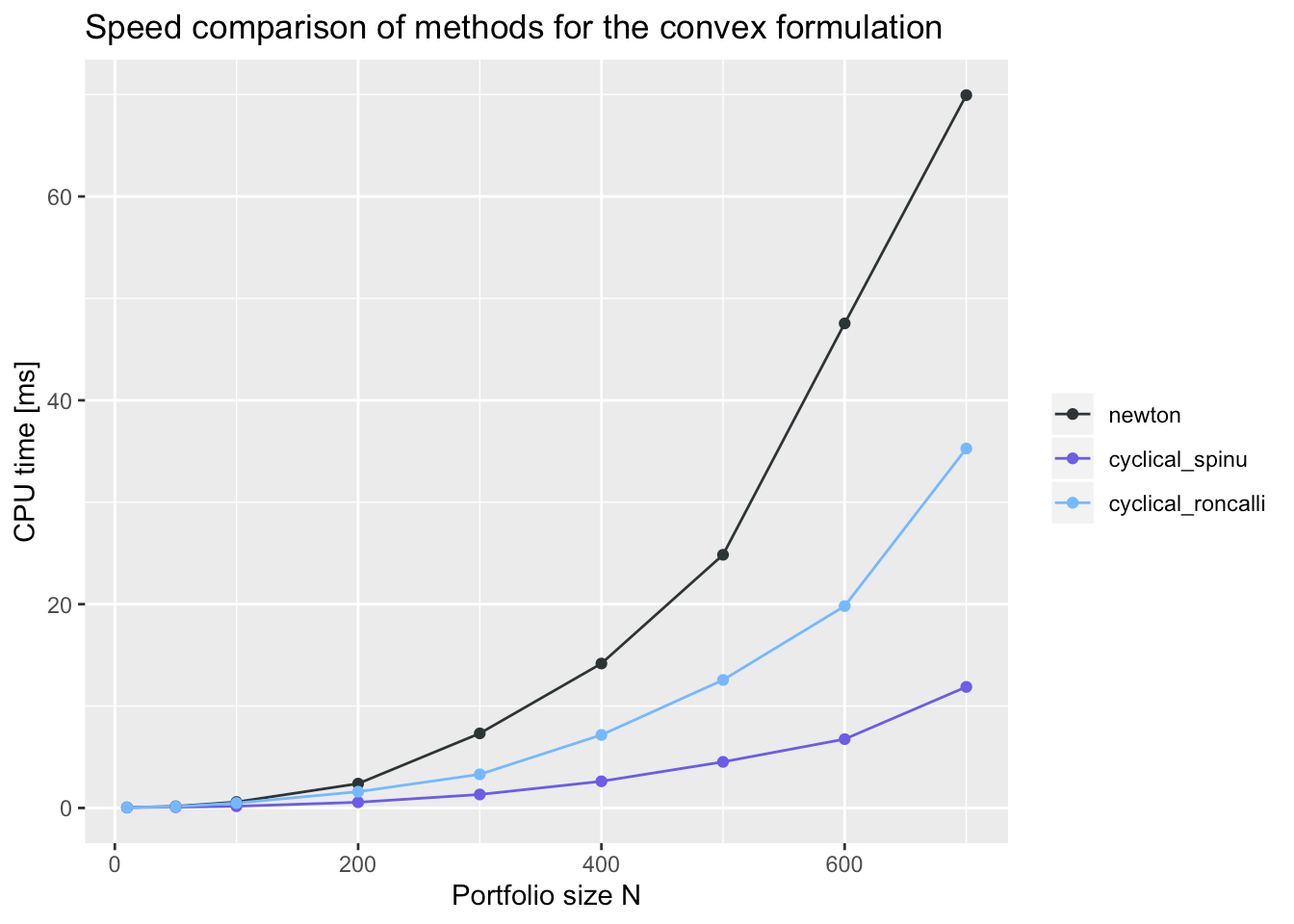# Fast Design of Risk Parity Portfolios

### 2019-10-05

This vignette illustrates the design of risk parity portfolios, which are widely used by practitioners in the financial industry, with the package riskParityPortfolio, giving a description of the algorithms and comparing the performance against existing packages.

# Quick Start

library(riskParityPortfolio)
?riskParityPortfolio  # to get help for the function

The simplest use is for the vanilla risk parity portfolio:

rpp_vanilla <- riskParityPortfolio(Sigma)
names(rpp_vanilla)
#>  "w"                          "relative_risk_contribution" "is_feasible"
round(rpp_vanilla$w, digits = 3) #> AAPL AMD ADI ABBV AEZS A APD AA CF #> 0.157 0.068 0.125 0.133 0.045 0.129 0.158 0.085 0.101 To obtain the naive diagonal solution, also known as inverse volatility portfolio, make use of the formulation argument: rpp_naive <- riskParityPortfolio(Sigma, formulation = "diag") For a more realistic formulation including the expected return in the objective and box constraints: $\begin{array}{ll} \underset{\mathbf{w}}{\textsf{minimize}} & \sum_{i,j=1}^{N}\left(\dfrac{w_{i}\left(\boldsymbol{\Sigma}\mathbf{w}\right)_{i}}{b_i}-\dfrac{w_{j}\left(\boldsymbol{\Sigma}\mathbf{w}\right)_{j}}{b_j}\right)^{2} \color{blue}{- \lambda \;\mathbf{w}^T\boldsymbol{\mu}}\\ \textsf{subject to} & \mathbf{w} \ge \mathbf{0}, \quad\mathbf{1}^T\mathbf{w}=1, \quad\color{blue}{\mathbf{l}\le\mathbf{w}\le\mathbf{u}}. \end{array}$ rpp_mu <- riskParityPortfolio(Sigma, formulation = "rc-over-b-double-index", mu = mu, lmd_mu = 1e-3, # for expected return term w_ub = 0.16) # for box upper bound constraints To plot and compare the results: w_all <- cbind("EWP" = rep(1/nrow(Sigma), nrow(Sigma)), "RPP (naive)" = rpp_naive$w,
"RPP (vanilla)" = rpp_vanilla$w, "RPP + mu" = rpp_mu$w)
barplotPortfolioRisk(w_all, Sigma)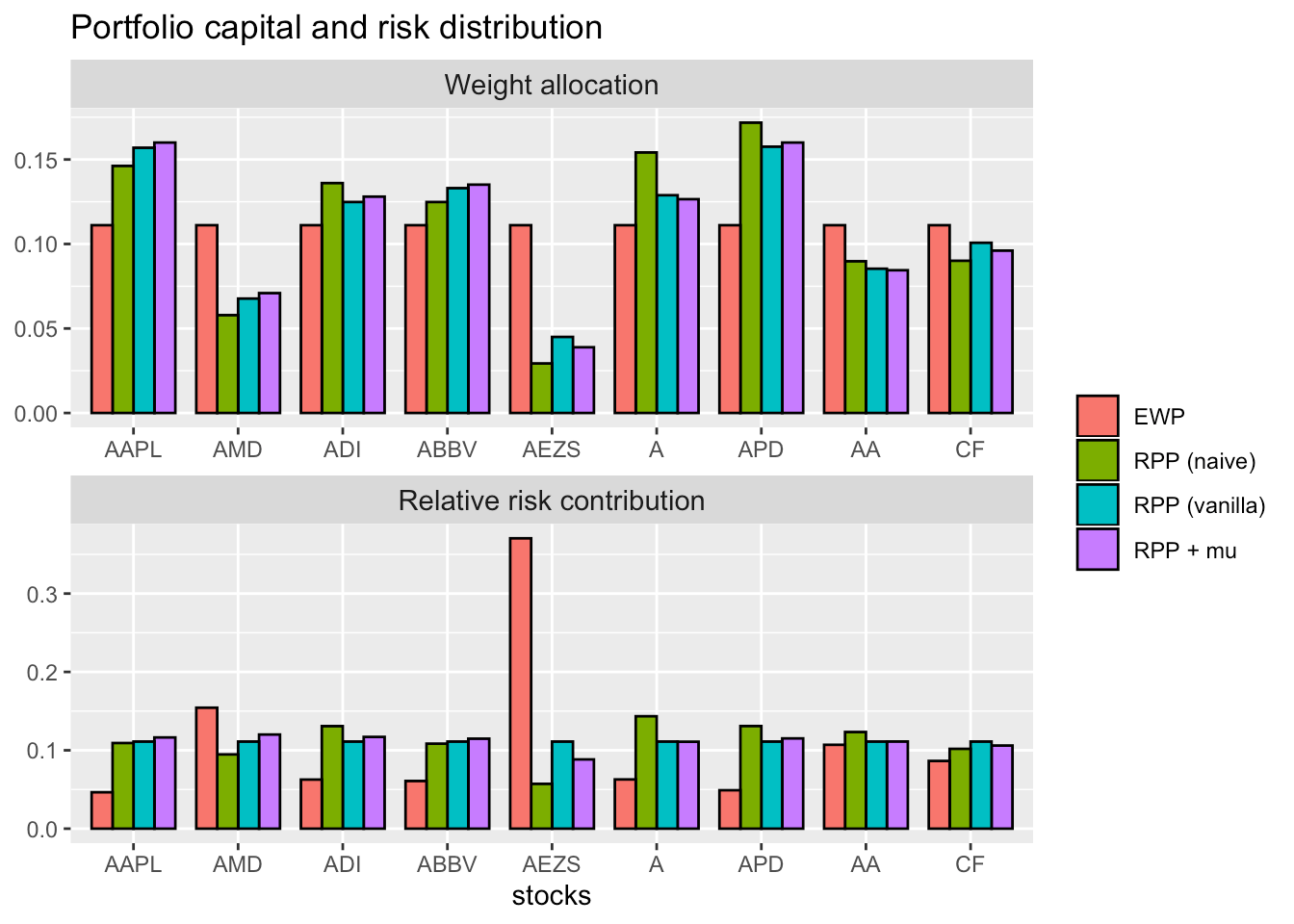# What is a Risk Parity Portfolio?

## Signal model

Let us denote by $$\mathbf{r}_{t}$$ the vector of the returns of $$N$$ assets at time $$t$$ and suppose they follow an i.i.d. distribution (which is not a totally accurate assumption, but it is widely adopted, nonetheless) with mean $$\boldsymbol{\mu}$$ and covariance matrix $$\boldsymbol{\Sigma}$$.

The portfolio vector $$\mathbf{w}$$ denotes the normalized dollar weights allocated to the $$N$$ assets, such that $$\mathbf{1}^{T}\mathbf{w}=1$$, and the portfolio return is then $$r_{t}^{\sf portf} = \mathbf{w}^{T}\mathbf{r}_{t}$$, with expected return $$\mathbf{w}^{T}\boldsymbol{\mu}$$ and variance $$\mathbf{w}^{T}\boldsymbol{\Sigma}\mathbf{w}$$.

## Modern Portfolio Theory

In 1952, Markowitz proposed in his seminal paper  to find a trade-off between the portfolio expected return and its risk measured by the variance: $\begin{array}{ll} \underset{\mathbf{w}}{\textsf{maximize}} & \mathbf{w}^{T}\boldsymbol{\mu}-\lambda\mathbf{w}^{T}\boldsymbol{\Sigma}\mathbf{w}\\ \textsf{subject to} & \mathbf{w} \ge \mathbf{0}, \quad\mathbf{1}^T\mathbf{w}=1, \end{array}$ where $$\lambda$$ is a parameter that controls how risk-averse the investor is.

Markowitz’s portfolio has been heavily critized for over half a century and has never been fully embraced by practitioners, among many reasons because:

• it only considers the risk of the portfolio as a whole and ignores the risk diversification (i.e., concentrates too much risk in few assets, which was observed in the 2008 financial crisis)
• it is highly sensitive to the estimation errors in the parameters (i.e., small estimation errors in the parameters may change the designed portfolio drastically).

Although portfolio management did not change much during the 40 years after the seminal works of Markowitz and Sharpe, the development of risk budgeting techniques marked an important milestone in deepening the relationship between risk and asset management.

## From “dollar” to risk diversification

Since the global financial crisis in 2008, risk management has particularly become more important than performance management in portfolio optimization. Indeed, risk parity became a popular financial model after the global financial crisis in 2008 , .

The alternative risk parity portfolio design has been receiving significant attention from both the theoretical and practical sides ,  because it: (1) diversifies the risk, instead of the capital, among the assets and (2) is less sensitive to parameter estimation errors.

Nowadays, pension funds and institutional investors are using this approach in the development of smart indexing and the redefinition of long-term investment policies.

Risk parity is an approach to portfolio management that focuses on allocation of risk rather than allocation of capital. The risk parity approach asserts that when asset allocations are adjusted to the same risk level, the portfolio can achieve a higher Sharpe ratio and can be more resistant to market downturns.

While the minimum variance portfolio tries to minimize the variance (with the disadvantage that a few assets may be the ones contributing most to the risk), the risk parity portfolio tries to constrain each asset (or asset class, such as bonds, stocks, real estate, etc.) to contribute equally to the portfolio overall volatility.

The term “risk parity” was coined by Edward Qian from PanAgora Asset Management  and was then adopted by the asset management industry. Some of its theoretical components were developed in the 1950s and 1960s, but the first risk parity fund, called the “All Weather” fund, was pioneered by Bridgewater Associates LP in 1996. The interest in the risk parity approach has increased since the financial crisis in the late 2000s as the risk parity approach fared better than portfolios designed in traditional fashions.

Some portfolio managers have expressed skepticism with risk parity, while others point to its performance during the financial crisis of 2007-2008 as an indication of its potential success.

The idea of the risk parity portfolio (RPP), aka equal risk portfolio (ERP), is to “equalize” the risk so that the risk contribution of every asset is equal, rather than simply having an equal capital allocation like the equally weighted portfolio (EWP):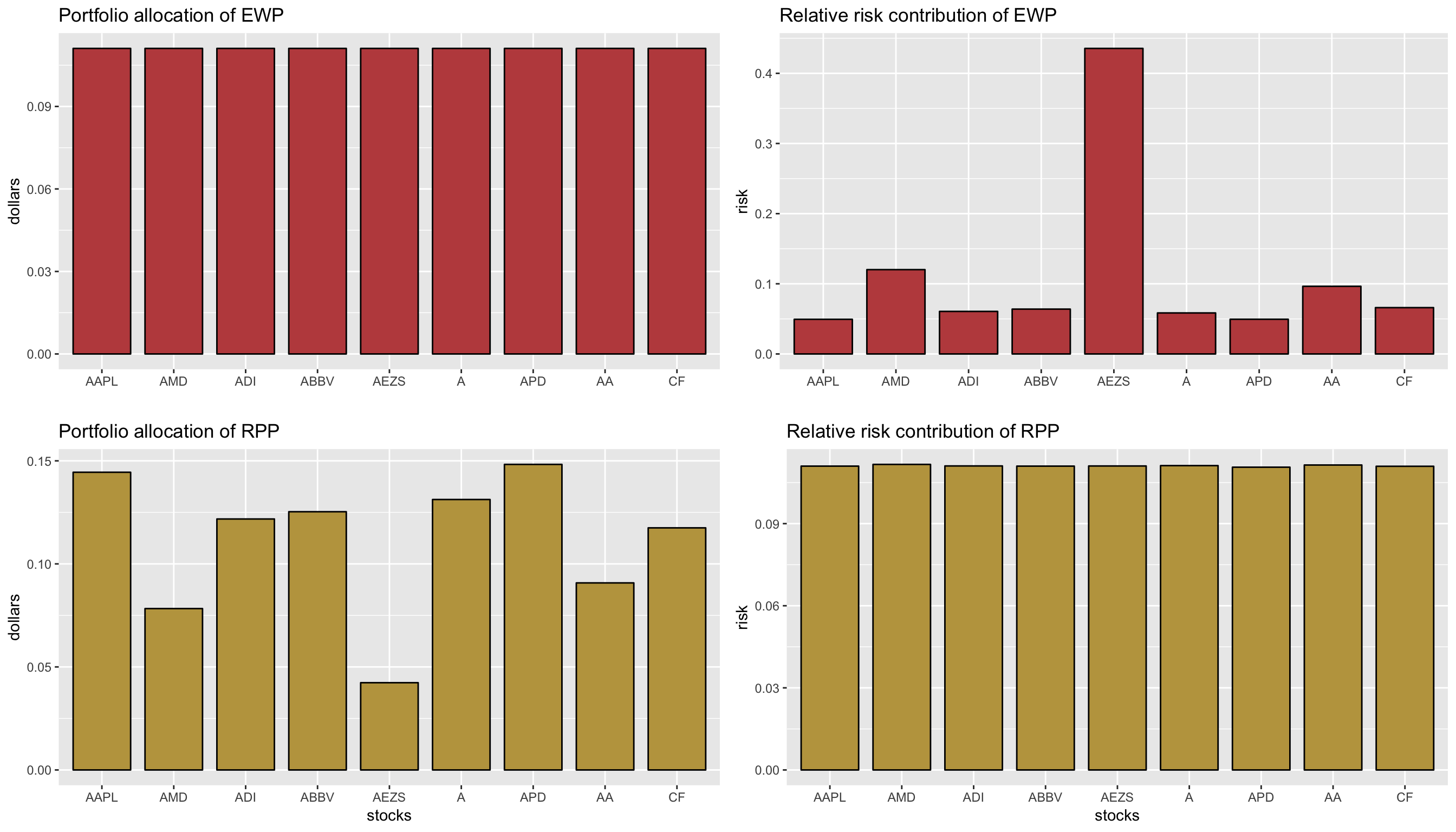## Risk parity portfolio

From Euler’s theorem, the volatility of the portfolio $$\sigma\left(\mathbf{w}\right)=\sqrt{\mathbf{w}^{T}\boldsymbol{\Sigma}\mathbf{w}}$$ can be decomposed as $\sigma\left(\mathbf{w}\right)=\sum_{i=1}^{N}w_i\frac{\partial\sigma}{\partial w_i} = \sum_{i=1}^N\frac{w_i\left(\boldsymbol{\Sigma}\mathbf{w}\right)_{i}}{\sqrt{\mathbf{w}^{T}\boldsymbol{\Sigma}\mathbf{w}}}.$

The risk contribution (RC) from the $$i$$th asset to the total risk $$\sigma(\mathbf{w})$$ is defined as ${\sf RC}_i =\frac{w_i\left(\boldsymbol{\Sigma}\mathbf{w}\right)_i}{\sqrt{\mathbf{w}^{T}\boldsymbol{\Sigma}\mathbf{w}}}$ which satisfies $$\sum_{i=1}^{N}{\sf RC}_i=\sigma\left(\mathbf{w}\right)$$.

The relative risk contribution (RRC) is a normalized version: ${\sf RRC}_i = \frac{w_i\left(\boldsymbol{\Sigma}\mathbf{w}\right)_i}{\mathbf{w}^{T}\boldsymbol{\Sigma}\mathbf{w}}$ so that $$\sum_{i=1}^{N}{\sf RRC}_i=1$$.

The risk parity portfolio (RPP) attemps to “equalize” the risk contributions: ${\sf RC}_i = \frac{1}{N}\sigma(\mathbf{w})\quad\text{or}\quad{\sf RRC}_i = \frac{1}{N}.$

More generally, the risk budgeting portfolio (RBP) attemps to allocate the risk according to the risk profile determined by the weights $$\mathbf{b}$$ (with $$\mathbf{1}^T\mathbf{b}=1$$ and $$\mathbf{b}\ge \mathbf{0}$$): ${\sf RC}_i = b_i \sigma(\mathbf{w})\quad\text{or}\quad{\sf RRC}_i = b_i.$

In practice, one can express the condition $${\sf RC}_i = \frac{1}{N}\sigma(\mathbf{w})$$ in different equivalent ways such as $w_i(\Sigma \mathbf{w})_{i} = w_j(\Sigma \mathbf{w})_{j}, \quad\forall i, j.$ The budget condition $${\sf RC}_i = b_i \sigma(\mathbf{w})$$ can also be expressed as $w_i (\Sigma \mathbf{w})_i = b_i \mathbf{w}^{T}\Sigma\mathbf{w}, \quad\forall i.$

# Solving the Risk Parity Portfolio (RPP)

## Naive diagonal formulation

Assuming that the assets are uncorrelated, i.e., that $$\boldsymbol{\Sigma}$$ is diagonal, and simply using the volatilities $$\boldsymbol{\sigma} = \sqrt{{\sf diag(\boldsymbol{\Sigma})}}$$, one obtains $\mathbf{w} = \frac{\boldsymbol{\sigma}^{-1}}{\mathbf{1}^T\boldsymbol{\sigma}^{-1}}$ or, more generally, $\mathbf{w} = \frac{\sqrt{\mathbf{b}}\odot\boldsymbol{\sigma}^{-1}}{\mathbf{1}^T\left(\sqrt{\mathbf{b}}\odot\boldsymbol{\sigma}^{-1}\right)}.$ However, for non-diagonal $$\boldsymbol{\Sigma}$$ or with other additional constraints or objective function terms, a closed-form solution does not exist and some optimization procedures have to be constructed. The previous diagonal solution can always be used and is called naive risk budgeting portfolio.

## Vanilla convex formulation

Suppose we only have the constraints $$\mathbf{1}^T\mathbf{w}=1$$ and $$\mathbf{w} \ge \mathbf{0}$$. Then, after the change of variable $$\mathbf{x}=\mathbf{w}/\sqrt{\mathbf{w}^{T}\boldsymbol{\Sigma}\mathbf{w}}$$, the equations $$w_i (\Sigma \mathbf{w})_i = b_i \mathbf{w}^{T}\Sigma\mathbf{w}$$ become $$x_i\left(\boldsymbol{\Sigma}\mathbf{x}\right)_i = b_i$$ or, more compactly in vector form, as $\boldsymbol{\Sigma}\mathbf{x} = \mathbf{b}/\mathbf{x}$ with $$\mathbf{x} \ge \mathbf{0}$$ and we can always recover the portfolio by normalizing: $$\mathbf{w} = \mathbf{x}/(\mathbf{1}^T\mathbf{x})$$.

At this point, one could use a nonlinear multivariate root finder for $$\boldsymbol{\Sigma}\mathbf{x} = \mathbf{b}/\mathbf{x}$$. For example, in R we can use the package rootSolve.

With the goal of designing risk budget portfolios, Spinu proposed in  to solve the following convex optimization problem: $\underset{\mathbf{x}\ge\mathbf{0}}{\textsf{minimize}} \quad \frac{1}{2}\mathbf{x}^{T}\boldsymbol{\Sigma}\mathbf{x} - \sum_{i=1}^{N}b_i\log(x_i),$ where the portfolio can be recovered as $$\mathbf{w} = \mathbf{x}/(\mathbf{1}^T\mathbf{x})$$.

Indeed, Spinu realized in  that precisely the risk budgeting equation $$\boldsymbol{\Sigma}\mathbf{x} = \mathbf{b}/\mathbf{x}$$ corresponds to the gradient of the convex function $$f(\mathbf{x}) = \frac{1}{2}\mathbf{x}^{T}\boldsymbol{\Sigma}\mathbf{x} - \mathbf{b}^T\log(\mathbf{x})$$ set to zero: $\nabla f(\mathbf{x}) = \boldsymbol{\Sigma}\mathbf{x} - \mathbf{b}/\mathbf{x} = \mathbf{0}.$

Thus, a convenient way to solve the problem is by solving the following convex optimization problem: $\underset{\mathbf{x}\ge\mathbf{0}}{\textsf{minimize}} \quad \frac{1}{2}\mathbf{x}^{T}\boldsymbol{\Sigma}\mathbf{x} - \mathbf{b}^T\log(\mathbf{x})$ which has optimality condition $$\boldsymbol{\Sigma}\mathbf{x} = \mathbf{b}/\mathbf{x}$$.

Such solution can be computed using a general-purpose convex optimization package, but faster algorithms such as the Newton method and the cyclical coordinate descent method, employed in  and , are implemented in this package. (Yet another convex formulation was proposed in .)

## General nonconvex formulation

The previous methods are based on a convex reformulation of the problem so they are guaranteed to converge to the optimal risk budgeting solution. However, they can only be employed for the simplest risk budgeting formulation with a simplex constraint set (i.e., $$\mathbf{1}^T\mathbf{w}=1$$ and $$\mathbf{w} \ge \mathbf{0}$$). They cannot be used if

• we have other constraints like allowing shortselling or box constraints: $$l_i \le w_i \le u_i$$
• on top of the risk budgeting constraints $$w_i\left(\boldsymbol{\Sigma}\mathbf{w}\right)_i = b_i \;\mathbf{w}^{T}\boldsymbol{\Sigma}\mathbf{w}$$ we have other objectives like maximizing the expected return $$\mathbf{w}^T\boldsymbol{\mu}$$ or minimizing the overall variance $$\mathbf{w}^T\boldsymbol{\Sigma}\mathbf{w}$$.

For those more general cases, we need more sophisticated formulations, which unfortunately are not convex. The idea is to try to achieve equal risk contributions $${\sf RC}_i = \frac{w_i\left(\boldsymbol{\Sigma}\mathbf{w}\right)_i}{\sqrt{\mathbf{w}^{T}\boldsymbol{\Sigma}\mathbf{w}}}$$ by penalizing the differences between the terms $$w_{i}\left(\boldsymbol{\Sigma}\mathbf{w}\right)_{i}$$.

There are many reformulations possible. For illustrative purposes, one such formulation is $\begin{array}{ll} \underset{\mathbf{w}}{\textsf{minimize}} & \sum_{i,j=1}^{N}\left(w_{i}\left(\boldsymbol{\Sigma}\mathbf{w}\right)_{i}-w_{j}\left(\boldsymbol{\Sigma}\mathbf{w}\right)_{j}\right)^{2} \color{blue}{- \;F(\mathbf{w})}\\ \textsf{subject to} & \mathbf{w} \ge \mathbf{0}, \quad\mathbf{1}^T\mathbf{w}=1, \quad\color{blue}{\mathbf{w}\in\cal{W}} \end{array}$ where $$F(\mathbf{w})$$ denotes some additional objective function and $$\cal{W}$$ denotes an arbitrary convex set of constraints. More expressions for the risk concentration terms are listed in Appendix I.

The way to solve this general problem is derived in ,  and is based on a powerful optimization framework named successive convex approximation (SCA) . See Appendix II for a general idea of the method.

# Using the Package riskParityPortfolio

A simple code example on how to design a risk parity portfolio is as follows:

library(riskParityPortfolio)

# generate synthetic data
set.seed(42)
N <- 10
V <- matrix(rnorm(N^2), nrow = N)
Sigma <- cov(V)

# compute risk parity portfolio
rpp <- riskParityPortfolio(Sigma)

# plot
risk_concentration <- c(risk_concentration, rpp$risk_concentration) } volatility <- sqrt(variance) ggplot(data.frame(risk_concentration, volatility), aes(x = risk_concentration, y = volatility)) + geom_line() + geom_point() + labs(title = "Volatility vs Risk Concentration", x = "Risk Concentration", y = "Volatility")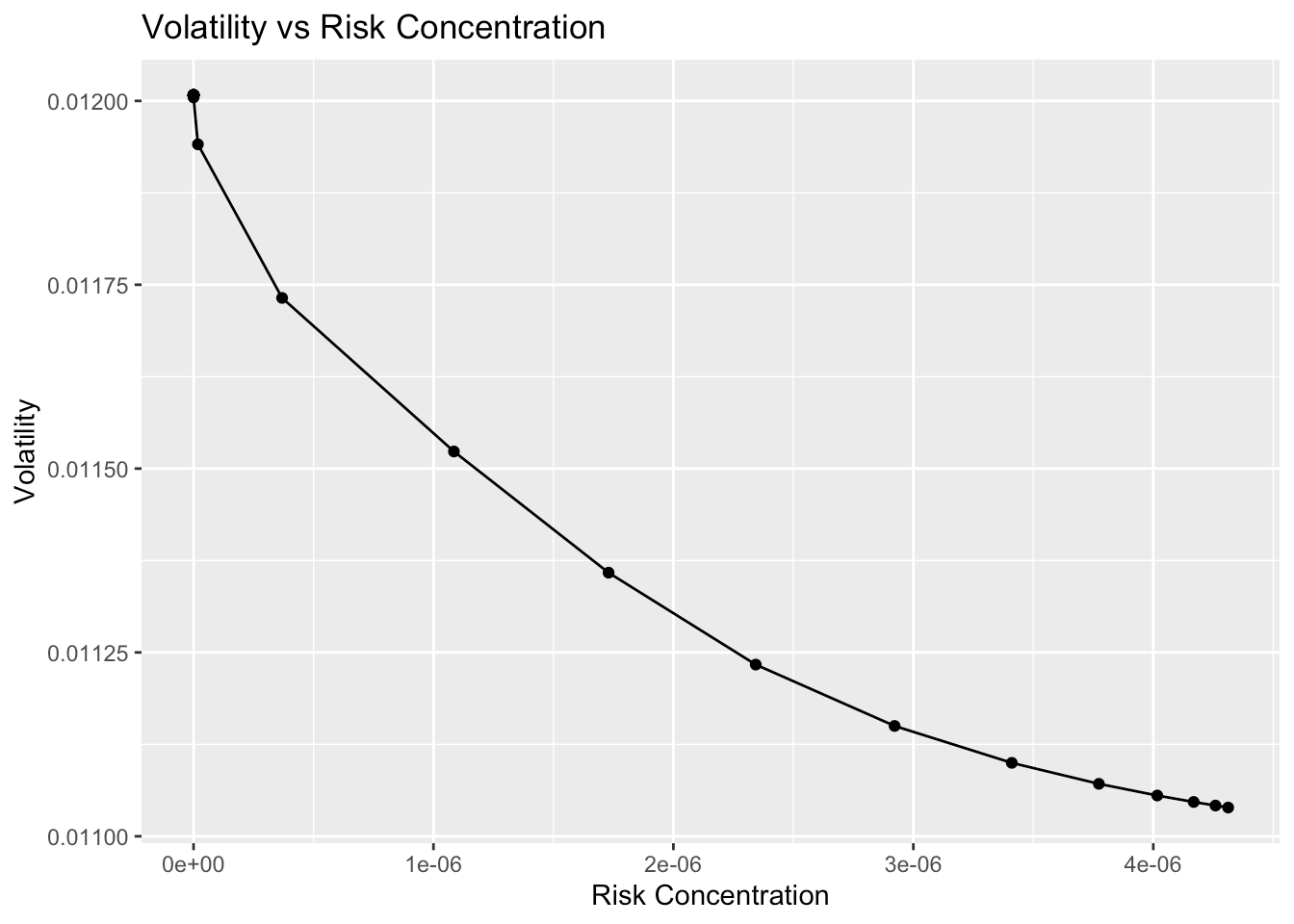## RPP with general linear constraints In version 2.0, we added support for general linear constraints, i.e., riskParityPortfolio is now able to solve the following problem: $\begin{array}{ll} \underset{\mathbf{w}}{\textsf{minimize}} & R(\mathbf{w}) + \lambda F(\mathbf{w})\\ \textsf{subject to} & \mathbf{C}\mathbf{w} = \mathbf{c},~~\mathbf{D}\mathbf{w} \leq \mathbf{d}. \end{array}$ Users interested in the details of the algorithm used to solve this problems are refered to Section V (Advanced Solving Approaches) of . In summary, the algorithm fits well within the SCA framework, while preserving speed and scalability. It was recently mentioned by  that the problem of designing risk parity portfolios with general constraints is harder than it seems. Indeed,  shows that, after imposing general linear constraints, the property of equal risk contributions (ERC) is unlikely to be preserved among the assets affected by the constraints. Let’s check out a numerical example from . Consider we have a universe of eight assets and we would like to design a risk parity portfolio $$\mathbf{w}$$ satisfying the following constraints: $w_5 + w_6 + w_7 + w_8 \geq 30\%,$ $w_2 + w_6 \geq w_1 + w_5 + 5\%,$ and $\sum_i w_i = 100\% .$ # compute the covariance matrix vol <- c(0.05, 0.05, 0.07, 0.1, 0.15, 0.15, 0.15, 0.18) Corr <- rbind(c(100, 80, 60, -20, -10, -20, -20, -20), c( 80, 100, 40, -20, -20, -10, -20, -20), c( 60, 40, 100, 50, 30, 20, 20, 30), c(-20, -20, 50, 100, 60, 60, 50, 60), c(-10, -20, 30, 60, 100, 90, 70, 70), c(-20, -10, 20, 60, 90, 100, 60, 70), c(-20, -20, 20, 50, 70, 60, 100, 70), c(-20, -20, 30, 60, 70, 70, 70, 100)) / 100 Sigma <- Corr * (vol %o% vol) # define linear constraints Dmat <- matrix(0, 2, 8) Dmat[1, ] <- c(rep(0, 4), rep(-1, 4)) Dmat[2, ] <- c(1, -1, 0, 0, 1, -1, 0, 0) dvec <- c(-0.30, -0.05) # design portfolio rpp <- riskParityPortfolio(Sigma, Dmat = Dmat, dvec = dvec) # plot portfolio weights barplotPortfolioRisk(rpp$w, Sigma)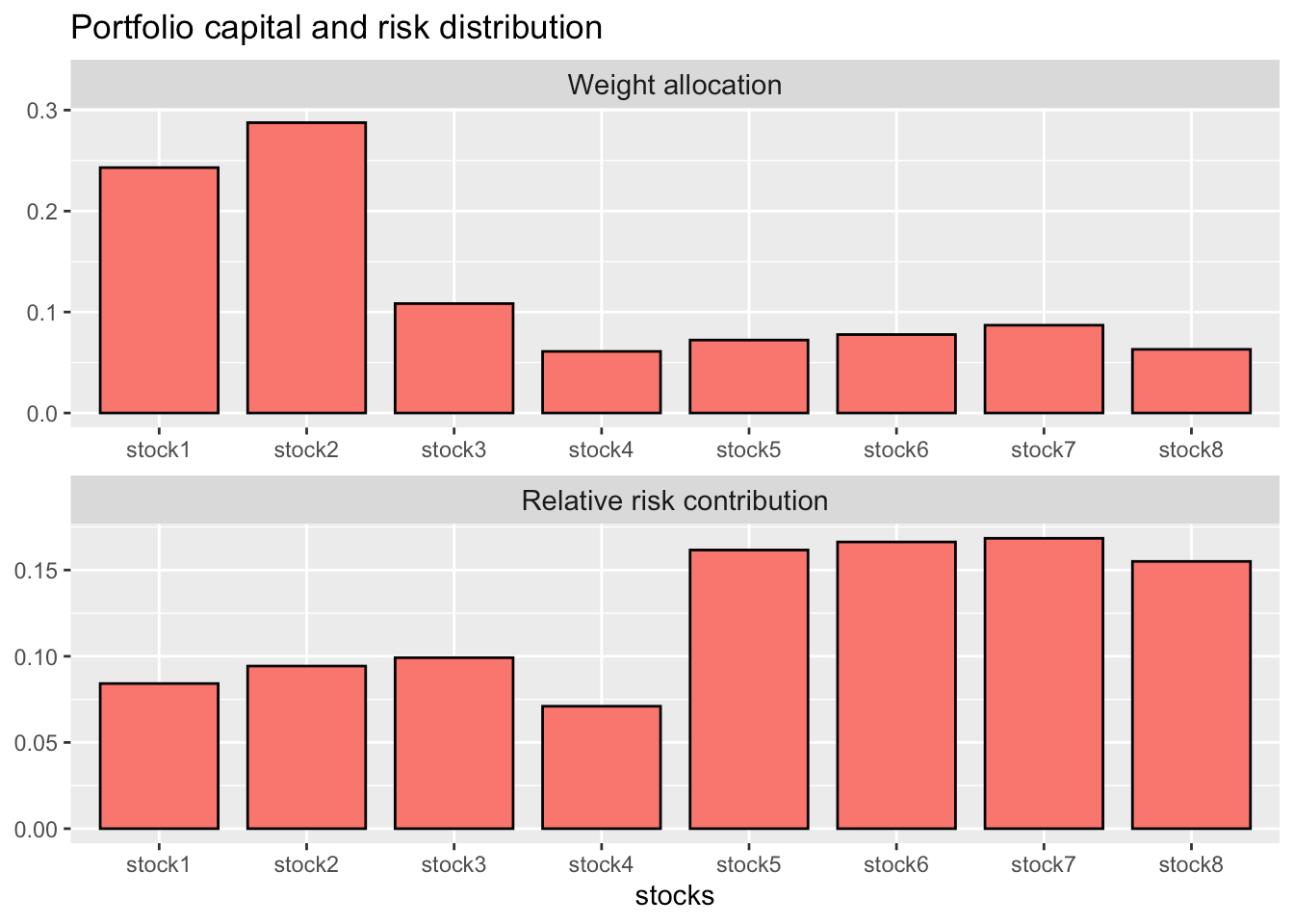As we can observe, the risk contributions are somewhat clustered according to the relationship among assets defined by the linear constraints, as mentioned by .

The linear constraints are obviously satisfied:

# equality constraints
print(sum(rpp$w)) #>  1 # inequality constraints print(Dmat %*% rpp$w)
#>       [,1]
#> [1,] -0.30
#> [2,] -0.05

The results obtained by our implementation agree with those reported by .

# A pratical example using FAANG price data

In , the author showed how to build a risk parity portfolio for FAANG companies (Facebook, Apple, Amazon, Netflix, and Google) using riskParityPortfolio. Here, we will attempt to replicate their backtest results, but using the package portfolioBacktest instead.

library(xts)
library(portfolioBacktest)
library(riskParityPortfolio)

from = "2014-01-01", to = "2019-06-25")

# define portfolios to be backtested
# risk parity portfolio
risk_parity <- function(dataset) {
prices <- dataset$adjusted log_returns <- diff(log(prices))[-1] return(riskParityPortfolio(cov(log_returns))$w)
}

# tangency portfolio (maximum sharpe ratio)
max_sharpe_ratio <- function(dataset) {
prices <- dataset$adjusted log_returns <- diff(log(prices))[-1] N <- ncol(prices) Sigma <- cov(log_returns) mu <- colMeans(log_returns) if (all(mu <= 1e-8)) return(rep(0, N)) Dmat <- 2 * Sigma Amat <- diag(N) Amat <- cbind(mu, Amat) bvec <- c(1, rep(0, N)) dvec <- rep(0, N) res <- solve.QP(Dmat = Dmat, dvec = dvec, Amat = Amat, bvec = bvec, meq = 1) w <- res$solution
return(w/sum(w))
}

# call portfolioBacktest and benchmark against the uniform (1/N) portfolio
bt <- portfolioBacktest(list("risk parity portfolio" = risk_parity,
"tangency portfolio"    = max_sharpe_ratio),
list(faang_data),
T_rolling_window = 12*20,
optimize_every = 3*20, rebalance_every = 3*20)

# dates of the designed portfolios
index(bt$tangency$data1$w_designed) #>  "2014-12-12" "2015-03-12" "2015-06-08" "2015-09-01" "2015-11-25" "2016-02-24" "2016-05-19" "2016-08-15" #>  "2016-11-08" "2017-02-06" "2017-05-03" "2017-07-28" "2017-10-23" "2018-01-19" "2018-04-17" "2018-07-12" #>  "2018-10-05" "2019-01-03" "2019-04-01" # check performance summary backtestSummary(bt)$performance
#>                   risk parity portfolio tangency portfolio
#> Sharpe ratio                  1.3800144          0.8787596
#> max drawdown                  0.3062046          0.3516856
#> annual return                 0.3117200          0.2324203
#> annual volatility             0.2258817          0.2644868
#> Sterling ratio                1.0180122          0.6608751
#> Omega ratio                   1.2710283          1.1793760
#> ROT (bps)                  8310.1199557        793.0188434

# plot cumulative returns chart
backtestChartCumReturns(bt)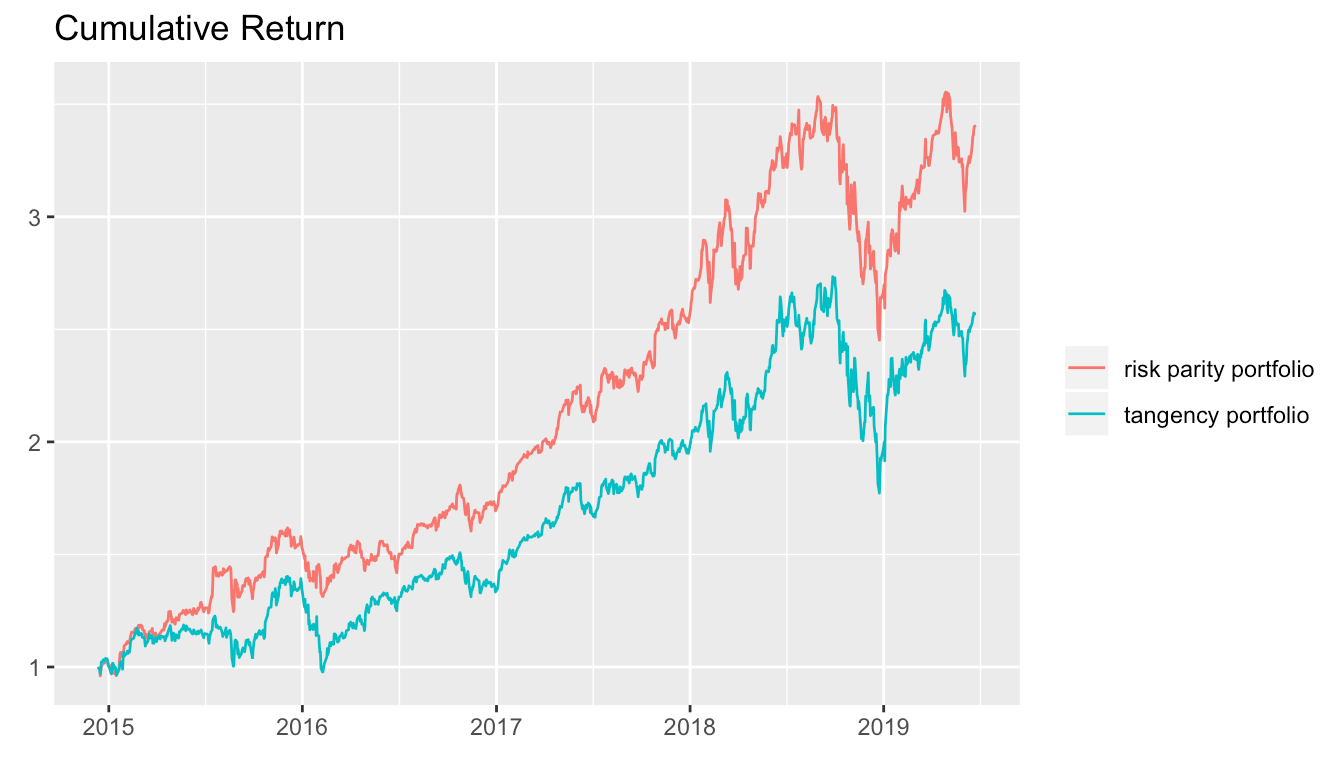# plot max drawdown chart
backtestChartDrawdown(bt)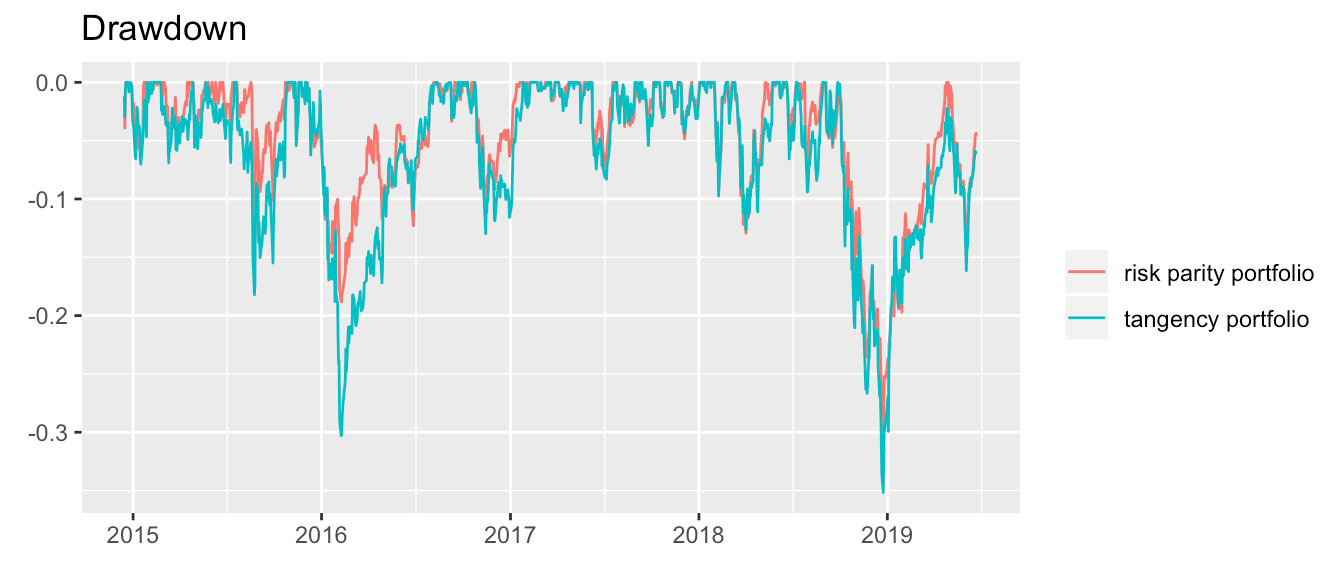# plot assets exposures over time
backtestChartStackedBar(bt, portfolio = "risk parity portfolio", legend = TRUE)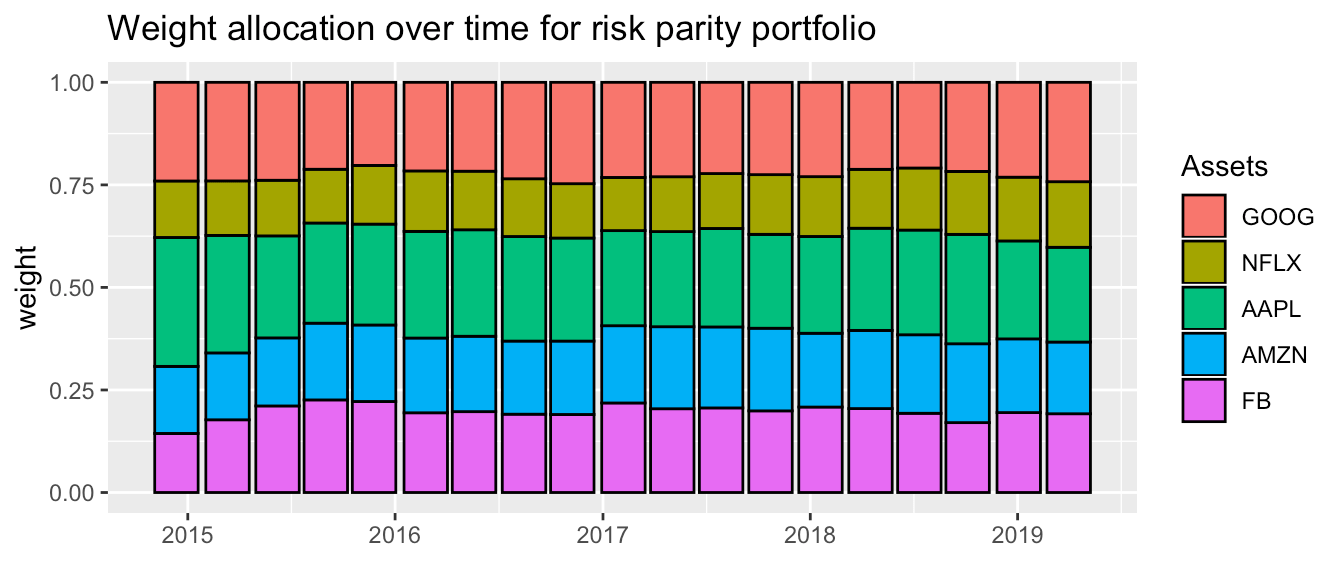backtestChartStackedBar(bt, portfolio = "tangency portfolio" , legend = TRUE)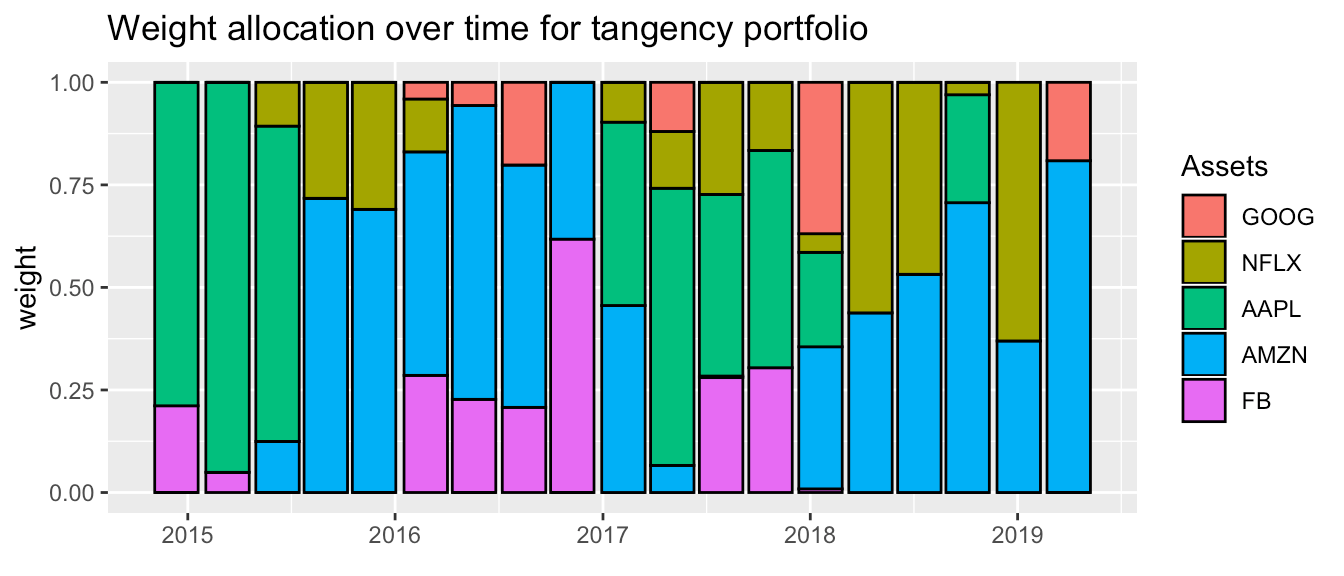Indeed, the charts are quite similar to those reported in . Note that the weights evolution of the Markowitz’s tangency portfolio is quite sensitive to minute changes in the rebalance/optimization dates, whereas no significant difference is noticed for the risk parity portfolio weights.

# Comparison with other Packages

Others R packages with the goal of designing risk parity portfolios do exist, such as FinCovRegularization, cccp, and RiskPortfolios. Let’s check how do they perform against riskParityPortfolio. (Note that other packages like FRAPO use cccp under the hood.)

library(FinCovRegularization)
library(cccp)
library(RiskPortfolios)

# generate synthetic data
set.seed(42)
N <- 10
V <- matrix(rnorm(N*(N+N/5)), N+N/5, N)
Sigma <- cov(V)

# uniform initial guess for the portfolio weights
w0 <- rep(1/N, N)

# compute risk parity portfolios using different methods
rpp <- riskParityPortfolio(Sigma, w0 = w0, formulation = "rc-double-index")
#> Warning in riskParityPortfolio(Sigma, w0 = w0, formulation = "rc-double-index"): The problem is a vanilla risk parity
#> portofolio, but a nonconvex formulation has been chosen. Consider not specifying the formulation argument in order to
#> use the convex formulation and get a guaranteed global solution.
fincov_w <- FinCovRegularization::RiskParity(Sigma)
riskport_w <- optimalPortfolio(Sigma = Sigma,
control = list(type = "erc",
constraint = "lo"))
cccp_w <- c(getx(cccp::rp(w0, Sigma, mrc = w0, optctrl = ctrl(trace = FALSE))))

# plot
w_all <- cbind("riskParityPortfolio"  = rppw, "FinCovRegularization" = fincov_w, "cccp" = cccp_w, "RiskPortfolios" = riskport_w) barplotPortfolioRisk(w_all, Sigma)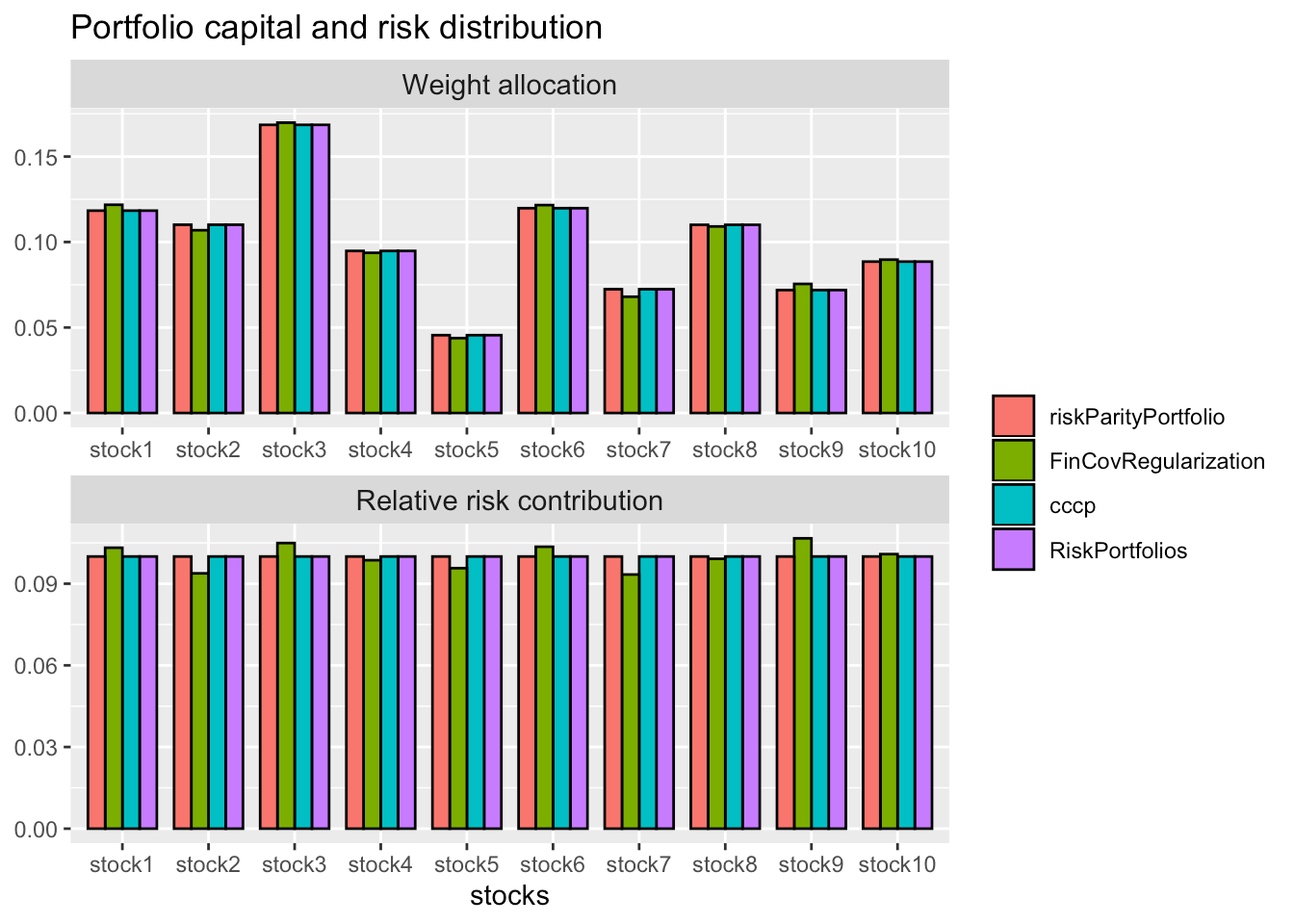Depending on the condition number of the covariance matrix, we found that the packages FinCovRegularization and RiskPortfolios may fail unexpectedly. Apart from that, all functions perform similarly. Now, let’s see a comparison, in terms of computational time, of our cyclical coordinate descent implementation against the rp() function from the cccp package and the optimalPortfolio() function from the RiskPortfolios package. (For a fair comparison, instead of calling our function riskParityPortfolio(), we call directly the core internal function risk_parity_portfolio_ccd_spinu(), which only computes the risk parity weights, just like rp() and optimalPortfolio().) library(microbenchmark) library(ggplot2) library(cccp) library(RiskPortfolios) library(riskParityPortfolio) N <- 100 V <- matrix(rnorm(N^2), ncol = N) Sigma <- cov(V) b <- rep(1/N, N) op <- microbenchmark( cccp = rp(b, Sigma, b, optctrl = ctrl(trace = FALSE)), riskParityPortfolio = riskParityPortfolio:::risk_parity_portfolio_ccd_spinu(Sigma, b, 1e-6, 50), RiskPortfolios = optimalPortfolio(Sigma = Sigma, control = list(type = 'erc', constraint = 'lo')), times = 10L) print(op) #> Unit: microseconds #> expr min lq mean median uq max neval #> cccp 15706.519 15907.386 17402.6694 16089.719 16160.337 29889.532 10 #> riskParityPortfolio 87.226 91.121 99.9547 96.808 108.079 119.291 10 #> RiskPortfolios 765344.494 769834.821 782709.4109 774997.356 790587.159 843189.970 10 autoplot(op) #> Coordinate system already present. Adding new coordinate system, which will replace the existing one.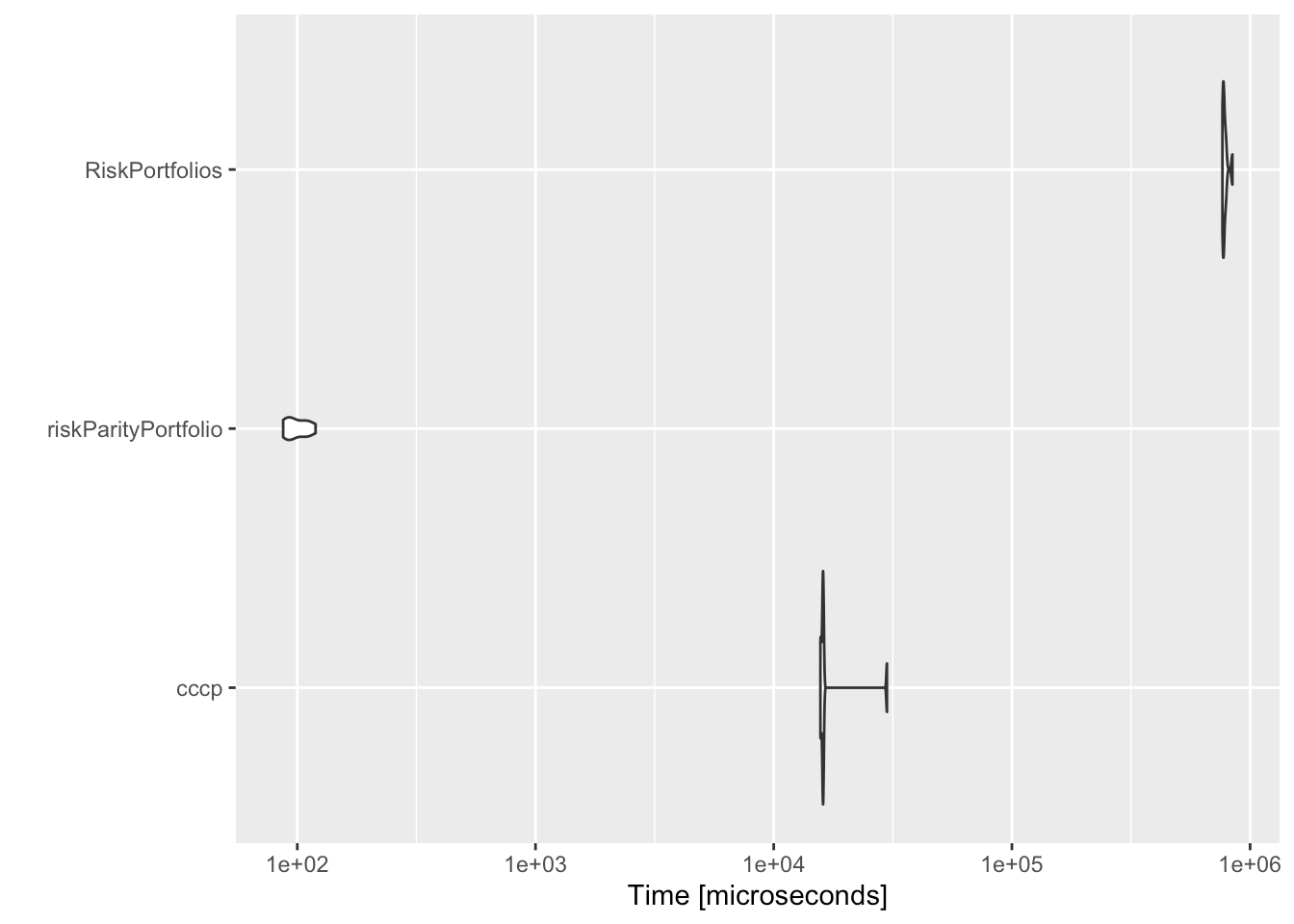As it can be observed, our implementation is orders of maginitude faster than the interior-point method used by cccp and the formulation used by RiskPortfolios. # Appendix I - Risk concentration formulations In general, with different constraints and objective functions, exact parity cannot be achieved and one needs to define a risk term to be minimized: $$R(\mathbf{w}) = \sum_{i=1}^{N}\left(g_{i}\left(\mathbf{w}\right)\right)^{2}$$, where the $$g_{i}$$’s denote the different risk contribution errors, e.g., $$g_{i} = w_{i}\left(\boldsymbol{\Sigma}\mathbf{w}\right)_i - b_i\mathbf{w}^T\boldsymbol{\Sigma}\mathbf{w}$$. A double-index summation can also be used: $$R(\mathbf{w}) = \sum_{i,j=1}^{N}\left(g_{ij}\left(\mathbf{w}\right)\right)^{2}$$. We consider the risk formulations as presented in , . They can be passed through the keyword argument formulation in the function riskParityPortfolio(). The name of the formulations and their mathematical expressions are presented as follows. Formulation “rc-double-index”: $R(\mathbf{w}) = \sum_{i,j=1}^{N}\left(w_{i}\left(\boldsymbol{\Sigma}\mathbf{w}\right)_{i}-w_{j}\left(\boldsymbol{\Sigma}\mathbf{w }\right)_{j}\right)^{2}$ Formulation “rc-vs-theta”: $R(\mathbf{w},\theta) = \sum_{i=1}^{N}\left(w_{i}\left(\boldsymbol{\Sigma}\mathbf{w}\right)_i - \theta \right)^{2}$ Formulation “rc-over-var-vs-b”: $R(\mathbf{w}) = \sum_{i=1}^{N}\left(\frac{w_{i}\left(\boldsymbol{\Sigma}\mathbf{w}\right)_i}{\mathbf{w}^T\boldsymbol{\Sigma}\mathbf{w}}-b_i\right)^{2}$ Formulation “rc-over-b double-index”: $R(\mathbf{w}) = \sum_{i,j=1}^{N}\left(\frac{w_i\left(\boldsymbol{\Sigma}\mathbf{w}\right)_i}{b_i} - \frac{w_j\left(\boldsymbol{\Sigma}\mathbf{w}\right)_j}{b_j}\right)^{2}$ Formulation “rc-vs-b-times-var”: $R(\mathbf{w}) = \sum_{i=1}^{N}\left(w_{i}\left(\boldsymbol{\Sigma}\mathbf{w}\right)_i - b_i\mathbf{w}^T\boldsymbol{\Sigma}\mathbf{w}\right)^{2}$ Formulation “rc-over-sd vs b-times-sd”: $R(\mathbf{w}) = \sum_{i=1}^{N}\left(\frac{w_{i}\left(\boldsymbol{\Sigma}\mathbf{w}\right)_i}{\sqrt{\mathbf{w}^T\boldsymbol{\Sigma}\mathbf{w}}}-b_i\sqrt{\mathbf{w}^T\boldsymbol{\Sigma}\mathbf{w}}\right)^{2}$ Formulation “rc-over-b vs theta”: $R(\mathbf{w},\theta) = \sum_{i=1}^{N}\left(\frac{w_{i}\left(\boldsymbol{\Sigma}\mathbf{w}\right)_i}{b_i} - \theta \right)^{2}$ Formulation “rc-over-var”: $R(\mathbf{w}) = \sum_{i=1}^{N}\left(\frac{w_{i}\left(\boldsymbol{\Sigma}\mathbf{w}\right)_i}{\mathbf{w}^T\boldsymbol{\Sigma}\mathbf{w}}\right)^{2}$ # Appendix II - Numerical algorithms for the risk parity portfolio In this appendix we describe the algorithms implemented for both the vanilla risk parity portfolio and the modern risk parity portfolio that may contain additional objective terms and constraints. ## Algorithms for the vanilla risk parity formulation We now describe the implementation of the Newton method and the cyclical (coordinate) descent algorithm for the vanilla risk parity formulations presented in  and . Consider the risk budgeting equations $w_i\left(\boldsymbol{\Sigma}\mathbf{w}\right)_i = b_i \;\mathbf{w}^{T}\boldsymbol{\Sigma}\mathbf{w}, \qquad i=1,\ldots,N$ with $$\mathbf{1}^T\mathbf{w}=1$$ and $$\mathbf{w} \ge \mathbf{0}$$. If we define $$\mathbf{x}=\mathbf{w}/\sqrt{\mathbf{w}^{T}\boldsymbol{\Sigma}\mathbf{w}}$$, then we can rewrite the risk budgeting equations compactly as $\boldsymbol{\Sigma}\mathbf{x} = \mathbf{b}/\mathbf{x}$ with $$\mathbf{x} \ge \mathbf{0}$$ and we can always recover the portfolio by normalizing: $$\mathbf{w} = \mathbf{x}/(\mathbf{1}^T\mathbf{x})$$. Spinu  realized that precisely that equation corresponds to the gradient of the function $$f(\mathbf{x}) = \frac{1}{2}\mathbf{x}^{T}\boldsymbol{\Sigma}\mathbf{x} - \mathbf{b}^T\log(\mathbf{x})$$ set to zero, which is the optimality condition for its minimization. So we can finally formulate the risk budgeting problem as the following convex optimization problem: $\underset{\mathbf{x}\ge\mathbf{0}}{\textsf{minimize}} \quad \frac{1}{2}\mathbf{x}^{T}\boldsymbol{\Sigma}\mathbf{x} - \mathbf{b}^T\log(\mathbf{x}).$ Roncalli et al.  proposed a slightly different formulation (also convex): $\underset{\mathbf{x}\ge\mathbf{0}}{\textsf{minimize}} \quad \sqrt{\mathbf{x}^{T}\boldsymbol{\Sigma}\mathbf{x}} - \mathbf{b}^T\log(\mathbf{x}).$ Unfortunately, even though these two problems are convex, they do not conform with the typical classes that most solvers embrace (i.e., LP, QP, QCQP, SOCP, SDP, GP, etc.). Nevertheless, there are several simple iterative algorithms that can be used, like the Newton method and the cyclical coordinate descent algorithm. Newton method The Newton method obtains the iterates based on the gradient $$\nabla f$$ and the Hessian $${\sf H}$$ of the objective function $$f(\mathbf{x})$$ as follows: $\mathbf{x}^{(k+1)} = \mathbf{x}^{(k)} - {\sf H}^{-1}(\mathbf{x}^{(k)})\nabla f(\mathbf{x}^{(k)})$ In practice, one may need to use the backtracking method to properly adjust the step size of each iteration . • For the function $$f(\mathbf{x}) = \frac{1}{2}\mathbf{x}^{T}\boldsymbol{\Sigma}\mathbf{x} - \mathbf{b}^T\log(\mathbf{x})$$, the gradient and Hessian are given by $\begin{array}{ll} \nabla f(\mathbf{x}) &= \boldsymbol{\Sigma}\mathbf{x} - \mathbf{b}/\mathbf{x}\\ {\sf H}(\mathbf{x}) &= \boldsymbol{\Sigma} + {\sf Diag}(\mathbf{b}/\mathbf{x}^2). \end{array}$ • For the function $$f(\mathbf{x}) = \sqrt{\mathbf{x}^{T}\boldsymbol{\Sigma}\mathbf{x}} - \mathbf{b}^T\log(\mathbf{x})$$, the gradient and Hessian are given by $\begin{array}{ll} \nabla f(\mathbf{x}) &= \boldsymbol{\Sigma}\mathbf{x}/\sqrt{\mathbf{x}^{T}\boldsymbol{\Sigma}\mathbf{x}} - \mathbf{b}/\mathbf{x}\\ {\sf H}(\mathbf{x}) &= \left(\boldsymbol{\Sigma} - \boldsymbol{\Sigma}\mathbf{x}\mathbf{x}^T\boldsymbol{\Sigma}/\mathbf{x}^{T}\boldsymbol{\Sigma}\mathbf{x}\right) / \sqrt{\mathbf{x}^{T}\boldsymbol{\Sigma}\mathbf{x}} + {\sf Diag}(\mathbf{b}/\mathbf{x}^2). \end{array}$ Cyclical coordinate descent algorithm This method simply minimizes in a cyclical manner with respect to each element of the variable $$\mathbf{x}$$ (denote $$\mathbf{x}_{-i}=[x_1,\ldots,x_{i-1},0,x_{i+1},\ldots,x_N]^T$$), while helding the other elements fixed. • For the function $$f(\mathbf{x}) = \frac{1}{2}\mathbf{x}^{T}\boldsymbol{\Sigma}\mathbf{x} - \mathbf{b}^T\log(\mathbf{x})$$, the minimization w.r.t. $$x_i$$ is $\underset{x_i>0}{\textsf{minimize}} \quad \frac{1}{2}x_i^2\boldsymbol{\Sigma}_{ii} + x_i(\mathbf{x}_{-i}^T\boldsymbol{\Sigma}_{\cdot,i}) - b_i\log{x_i}$ with gradient $$\nabla_i f = x_i\boldsymbol{\Sigma}_{ii} + (\mathbf{x}_{-i}^T\boldsymbol{\Sigma}_{\cdot,i}) - b_i/x_i$$. Setting the gradient to zero gives us the second order equation $x_i^2\boldsymbol{\Sigma}_{ii} + x_i(\mathbf{x}_{-i}^T\boldsymbol{\Sigma}_{\cdot,i}) - b_i = 0$ with positive solution given by $x_i^\star = \frac{-(\mathbf{x}_{-i}^T\boldsymbol{\Sigma}_{\cdot,i})+\sqrt{(\mathbf{x}_{-i}^T\boldsymbol{\Sigma}_{\cdot,i})^2+ 4\boldsymbol{\Sigma}_{ii} b_i}}{2\boldsymbol{\Sigma}_{ii}}.$ • The derivation for the function $$f(\mathbf{x}) = \sqrt{\mathbf{x}^{T}\boldsymbol{\Sigma}\mathbf{x}} - \mathbf{b}^T\log(\mathbf{x})$$ follows similarly. The update for $$x_i$$ is given by $x_i^\star = \frac{-(\mathbf{x}_{-i}^T\boldsymbol{\Sigma}_{\cdot,i})+\sqrt{(\mathbf{x}_{-i}^T\boldsymbol{\Sigma}_{\cdot,i})^2+ 4\boldsymbol{\Sigma}_{ii} b_i \sqrt{\mathbf{x}^{T}\boldsymbol{\Sigma}\mathbf{x}}}}{2\boldsymbol{\Sigma}_{ii}}.$ ## Successive convex approximation algorithm for the modern risk parity formulation Many practical formulations deployed to design risk parity portfolios lead to nonconvex problems, specially when additional objective terms such as mean return or variance, or additional constraints, namely, shortselling, are taken into account. To circumvent the complications that arise in such formulations, Feng & Palomar  proposed a method called sucessive convex approximation (SCA). The SCA method works by convexifying the risk concentration term at some pre-defined point, casting the nonconvex problem into a much simpler strongly convex optimization problem. This procedure is then iterated until convergence is achieved. It is important to highlight that the SCA method always converges to a stationary point. At the $$k$$-th iteration, the SCA method aims to solve \begin{align}\begin{array}{ll} \underset{\mathbf{w}}{\textsf{minimize}} & \sum_{i=1}^{n}\left(g_i(\mathbf{w}^k) + (\nabla g_i(\mathbf{w}^{k}))^{T}(\mathbf{w} - \mathbf{w}^{k})\right)^2 + \tau ||\mathbf{w} - \mathbf{w}^{k}||^{2}_{2} + \lambda F(\mathbf{w})\\ \textsf{subject to} & \mathbf{w}^{T}\mathbf{1} = 1, \mathbf{w} \in \mathcal{W}, \end{array}\end{align} where the first order Taylor expasion of $$g_i(\mathbf{w})$$ has been used. After some mathematical manipulations described in detail in , the optimization problem above can be rewritten as \begin{align}\begin{array}{ll} \underset{\mathbf{w}}{\textsf{minimize}} & \dfrac{1}{2}\mathbf{w}^{T}\mathbf{Q}^{k}\mathbf{w} + \mathbf{w}^{T}\mathbf{q}^{k} + \lambda F(\mathbf{w})\\ \textsf{subject to} & \mathbf{w}^{T}\mathbf{1} = 1, \mathbf{w} \in \mathcal{W}, \end{array}\end{align} where \begin{align} \mathbf{Q}^{k} & \triangleq 2(\mathbf{A}^{k})^{T}\mathbf{A}^{k} + \tau \mathbf{I},\\ \mathbf{q}^{k} & \triangleq 2(\mathbf{A}^{k})^{T}\mathbf{g}(\mathbf{w}^{k}) - \mathbf{Q}^{k}\mathbf{w}^{k}, \end{align} and \begin{align} \mathbf{A}^{k} & \triangleq \left[\nabla_{\mathbf{w}} g_{1}\left(\mathbf{w}^{k}\right), ..., \nabla_{\mathbf{w}} g_{n}\left(\mathbf{w}^{k}\right)\right]^{T} \\ \mathbf{g}\left(\mathbf{w}^{k}\right) & \triangleq \left[g_{1}\left(\mathbf{w}^{k}\right), ..., g_{n}\left(\mathbf{w}^{k}\right)\right]^{T}. \end{align} The above problem is a quadratic program (QP) which can be efficiently solved by standard R libraries. Furthermore, it is straightforward that adding the mean return or variance terms still keeps the structure of the problem intact. # Appendix III - Computational time In order to efficiently design high dimensional portfolios that follows the risk parity criterion, we implement the cyclical coordinate descent algorithm proposed by . In brief, this algorithm optimizes for one portfolio weight at a time while leaving the rest fixed. The plot below illustrates the computational scaling of both Newton and cyclical algorithms. Note that the cyclical algorithm is implemented for two different formulations used by  and , respectively. Nonetheless, they output the same solution, as they should. library(microbenchmark) library(riskParityPortfolio) library(ggplot2) sizes <- c(10, 50, 100, 200, 300, 400, 500, 600, 700) size_seq <- c(1:length(sizes)) times <- matrix(0, 3, length(sizes)) for (i in size_seq) { V <- matrix(rnorm(1000 * sizes[i]), nrow = sizes[i]) Sigma <- V %*% t(V) bench <- microbenchmark( newton = riskParityPortfolio(Sigma, method_init="newton"), cyclical_spinu = riskParityPortfolio(Sigma, method_init="cyclical-spinu"), cyclical_roncalli = riskParityPortfolio(Sigma, method_init="cyclical-roncalli"), times = 10L, unit = "ms", control = list(order = "inorder", warmup = 4)) times[1, i] <- median(benchtime[c(TRUE, FALSE, FALSE)] / 10 ^ 6)
times[2, i] <- median(bench$time[c(FALSE, TRUE, FALSE)] / 10 ^ 6) times[3, i] <- median(bench$time[c(FALSE, FALSE, TRUE)] / 10 ^ 6)
}

df <- data.frame(sizes, newton = times[1, ], cyclical_spinu = times[2, ], cyclical_roncalli = times[3, ])
molten_df <- reshape2::melt(df, id.vars = "sizes")
ggplot(molten_df, aes(x = sizes, y = value, col = variable)) +
geom_line() + geom_point() +
scale_color_manual(values = c("#2d3436", "#6c5ce7", "#74b9ff")) +
theme(legend.title = ggplot2::element_blank()) +
labs(title = "Speed comparison of methods for the convex formulation",
x = "Portfolio size N", y = "CPU time [ms]")